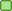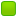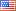###Author Topic: Comparing the input with integer values  (Read 13876 times)

####animefuns

• New Member
• Posts: 1##### Comparing the input with integer values
« on: April 01, 2012, 08:37:32 PM »
I am trying to compare an input data with a integer value

here the basic code I am using

Code: [Select]
`section .bss    input resb 2section .textglobal _start_start:    mov eax, 3    mov ebx, 1    mov ecx, input    mov edx, 5    int 0x80    mov eax, [input]    cmp eax, 20 ; This is what I cannot get to work, it never compares it to 20 even if i enter 20 as input    je next`
All I really want to know is how to do the If statement in Assembly to compare input with an integer.

I would really apprecaite any help with this, thank you.

####Frank Kotler

• NASM Developer
• Hero Member
•• Posts: 2629
• Country:##### Re: Comparing the input with integer values
« Reply #1 on: April 01, 2012, 11:46:14 PM »
Well, your basic problem is that "input" is characters. These characters are integers, of course, but they aren't the integers the characters represent. For example, if the user enters "20" (which I guess is what you're looking for), what's in the buffer is 0x32 ("2"), 0x30 ("0")... and a linefeed (0xA) 'cause sys_read doesn't return until it sees one.

Code: [Select]
`section .bss    input resb 2section .textglobal _start_start:    mov eax, 3    mov ebx, 1    mov ecx, input    mov edx, 5    int 0x80`Whoa! You've only reserved two bytes for your buffer and allowed the user to put (up to) five bytes into it. That's a "buffer overflow"! Very bad! Won't do any harm in this case, since there's nothing after your buffer, but don't do it! How many characters do you actually want to allow the user to enter? A full 32-bit integer will take (up to) 10 characters to represent. You probably want one extra to hold the linefeed. You may want to allow a minus sign, if you're going to handle signed integers. So you want to reserve (at least) that many bytes in your input buffer.

If the user keeps typing more than what you've allowed in edx (up until "enter" is hit, remember), the excess stays in the OS's input buffer (won't overwrite your buffer -  edx bytes maximum), and will be read by the next sys_read - will end up on the command line, in this case. We really should flush the (OS's) input buffer at this point, but to "keep it simple" we can ignore that for now... (this is called "teaching sloppy solutions to beginners" - my bad!)

At this point, eax will contain the number of characters actually entered (including the linefeed). This can be useful to note/save, but we don't really need it.

Code: [Select]
`    mov eax, [input]`
The input buffer will hold, for this example, say 0x32, 0x30, 0xA. Putting this in eax (as 0x000A3032) is not too useful. What you want to do is get one character at a time, subtract '0' (0x30, 48 decimal) to convert it from a character to the number it represents, multiply a "result so far" by ten, and add in the number... until done.

Since we want the result in eax, we might as well use that as a "result so far" - zero it first. Since ecx already points to our input buffer, we might as well use that. We want a full 32-bit register to add to our "result so far", but we only want a byte in it, with the upper bits zeroed. "movzx" will do that, but we can do it another way...
Code: [Select]
`xor eax, eax  ; (or mov eax, 0) zero "result so far"xor ebx, ebx  ; zero upper bits of ebx for our charactermov esi, 10  ; for a multipliertop:mov bl, [ecx]  ; get a characterinc ecx  ; get ready for the next onecmp bl, '0'  ; is it a valid decimal digit?jb donecmp bl, '9'ja donemul esi  ; eax * esi -> edx:eaxadd eax, ebx ; valid digit - add to "result so far"jmp top  ; do anotherdone:; now you can compare integer to integer!    cmp eax, 20 ; This is what I cannot get to work, it never compares it to 20 even if i enter 20 as input    je next`
That's untested and probably has errors - I'm kinda distracted just now - but that's the general idea...

Best,
Frank

####codeFoil

• Jr. Member
•• Posts: 13##### Re: Comparing the input with integer values
« Reply #2 on: April 02, 2012, 06:23:32 AM »
Very distractedAnimeFuns,  as Frank has pointed out, the difficulty is that your program is attempting to perform three distinct tasks.  You want your program to accept character input, and then test for a decimal value represented by those characters.

This requires these steps:
1. Read a string of characters.
2. Converting that string of characters into a number.
3. Compare that number with another number.

The third step is trivial.  Getting there is not so simple.

Again, as Frank has indicated, the system call fills a memory buffer with byte values representing the characters entered, in the order they are entered.  He has also given an idea of how that works.
He then put you on the correct track to tackle the second step, where you will convert that string into a number.  That's when he must have gotten distracted.

There are a handful of way numbers may be represented in memory.  The easiest to work with are Integers, and between signed and unsigned Integers, unsigned Integers are simplest.

Converting the characters to Integer involves converting each character code value to a digit, and then multiplying each digit by a power of ten according to its position.  The conversion of the character code to a digit is pretty simply, because the ASCII codes for the digit characters are all in order.  We simply need to subtract the value of "0" (which is 0x30 in hexadecimal, 48 is decimal).

Multiplying each digit is pretty simple, but the problem is that we need to know the position of the digit starting from the RIGHT side of the string.  The first character in the memory buffer, however, is on the LEFT side of the string.

One approach is to begin by converting each character into a digit value and save that value on the stack, counting digits are we go.  When the conversion of each character is complete, we know how many digits we have and the LAST digit is ready to be popped off the stack.  Now, we can simply remove each digit from the stack and multiply it by a power of ten, starting with 1, and collect a running sum of the results.  After each digit, we multiply our multiplier by ten to get the next power of ten.

There are a few complications.  We have to take into account that if the string represents a number larger than 2^(32-1), our result will be incorrect due to overflow.  The other issue is that we must be absolutely certain that we leave the stack in the same condition that we found it.

Code: [Select]
`; This assumes that you have a string stored and have determined ; its maximum possible length.        MOV  EDI, string                 MOV  ECX, [maxLength]   ; Set loop counter        XOR  EBX, EBX           ; Use EBX to count characters        XOR  EAX, EAX           ; Clear all bits in EAX.ReadDigits        MOV  AL, byte [EDI]     ; Get a character        INC  EDI                ; Point to the next character        CMP  AL, '0'            ; Test for numerical        JB   .DigitsRead                    CMP  AL, '9'        JA   .DigitsRead        PUSH EAX                ; Place on stack        INC  EBX        LOOP .ReadDigits        ; Repeat.DigitsRead:                MOV  ECX, EBX           ; Set loop counter        XOR  EBX, EBX           ; Clear sum        TEST ECX, ECX           ; Test for no characters        JZ   .Done        MOV  ESI, 1             ; Set multiplier to 1.Convert:        POP  EAX                ; Get digit from stack        MUL  ESI                ; Multiply        TEST EDX, EDX           ; If EDX does not = 0, an overflow occurred        JNZ  .overflow        ADD  EBX, EAX           ; Accumulate result        jc   .Overflow          ; If carry flag is set, an overflow occured        MOV  EAX, 10            ; Use base 10 for decimal notation           MUL  ESI                ; Set multiplier to multiplier * base        MOV  ESI, EAX        LOOP .Convert        MOV  EAX, EBX           ; Using EAX to hold result        JMP  .Done.Overflow:        POP   EAX               ; cleanup stack        LOOP  .Overflow;   Here we'll just set the ;   value to maximum. A more ;   robust program would some;   how indicate that an error ;   occurred.    MOV   EAX, 0xFFFFFFFF.Done:;Integer value is now in EAX`

####Frank Kotler

• NASM Developer
• Hero Member
•• Posts: 2629
• Country:##### Re: Comparing the input with integer values
« Reply #3 on: April 02, 2012, 02:09:39 PM »
Yeah, very distracted. Left out a vital step: subtracting '0'! Thanks CodeFoil!

Best,
Frank

####codeFoil

• Jr. Member
•• Posts: 13##### Re: Comparing the input with integer values
« Reply #4 on: April 02, 2012, 07:39:02 PM »
Yeah, very distracted. Left out a vital step: subtracting '0'! Thanks CodeFoil!

Best,
Frank
I swear mine got left in the clip board!

Other wise, your approach is superior.  My intention was to break the task down into simpler steps.  Once each is understood, it's just one more step to interleave them to arrive at your code.  Shuffling around, stack storage is eliminated and the conversion can be done from first to last.
Code: [Select]
`; This assumes that you have a string stored and have determined ; its maximum possible length.                XOR  EAX, EAX           ; Set initial digit value to zero        MOV  ECX, [maxLength]   ; Set loop counter        TEXT ECX, ECX           ; check for no characters        JZ   .Done        MOV  EDI, string                 MOV  ESI, 10            ; Set base        XOR  EBX, EBX           ; Use EBX to accumulate sum.ReadandConvert:        MUL  EBX, ESI           ; Multiply current sum by base        MOV  EBX, EAX           ; and save        TEST EDX, EDX           ; Check for overflow        JNZ  .OverFlow                  XOR  EAX, EAX           ; Clear all bits in EAX        MOV  AL, byte [EDI]     ; Get a character        INC  EDI                ; Point to the next character        CMP  AL, '0'            ; Test for numerical        JB   .ReadAndConverted        CMP  AL, '9'        JA   .ReadAndConverted        SUB  AL, '0'            ; Adjust character code to digit value        ADD  EBX, EAX           ; and add to accumulated value        JC   .Overflow          ; Check for overflow        LOOP .ReadAndConvert ; Repeat        MOV  EAX, EBX        JMP .Done.ReadandConverted:.Overflow:;   Here we'll just set the ;   value to maximum. A more ;   robust program would some;   how indicate that an error ;   occurred.    MOV   EAX, 0xFFFFFFFF.Done:`